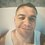# Python Solution for Perceptron Learning Algorithm (Artificial Neural Networks Course) Page 9 Problem

def vecsum(a,b):
return [e1 + e2 for e1,e2 in zip(a,b)]

def scalarproduct(c,a):
return [c*e for e in a]

def dotproduct(a,b):
return sum([e1*e2 for e1,e2 in zip(a,b)])

def perceptron(w,x,b):
out = dotproduct(w,x) + b
if out == 0:
return 0
elif out > 0:
return 1
elif out < 0:
return -1

def vecequal(a,b):
return all([e1 == e2 for e1,e2 in zip(a,b)])

b = 0
w = [0,0]
x = [(-1, 1),(0, -1),(10, 1)]
y = [1,-1,1]
outputs = [perceptron(w,e,b) for e in x]
size = len(y)
turn = 0

while not vecequal(y,outputs):
print(turn,w,b)
print(y,outputs)

outputs[turn] = perceptron(w,x[turn],b)
if  outputs[turn] != y[turn]:
w = vecsum(w,scalarproduct(y[turn],x[turn]))
b = b + y[turn]

turn = (turn + 1) % size
raw_input("Press Enter to continue...")

print("CONVERGENCE REACHED!")
print(w,b)
print(y,outputs)Note by Talles Brito
2 years ago

This discussion board is a place to discuss our Daily Challenges and the math and science related to those challenges. Explanations are more than just a solution — they should explain the steps and thinking strategies that you used to obtain the solution. Comments should further the discussion of math and science.

When posting on Brilliant:

• Use the emojis to react to an explanation, whether you're congratulating a job well done , or just really confused .
• Ask specific questions about the challenge or the steps in somebody's explanation. Well-posed questions can add a lot to the discussion, but posting "I don't understand!" doesn't help anyone.
• Try to contribute something new to the discussion, whether it is an extension, generalization or other idea related to the challenge.

MarkdownAppears as
*italics* or _italics_ italics
**bold** or __bold__ bold
- bulleted- list
• bulleted
• list
1. numbered2. list
1. numbered
2. list
Note: you must add a full line of space before and after lists for them to show up correctly
paragraph 1paragraph 2

paragraph 1

paragraph 2

[example link](https://brilliant.org)example link
> This is a quote
This is a quote
    # I indented these lines
# 4 spaces, and now they show
# up as a code block.

print "hello world"
# I indented these lines
# 4 spaces, and now they show
# up as a code block.

print "hello world"
MathAppears as
Remember to wrap math in $$ ... $$ or $ ... $ to ensure proper formatting.
2 \times 3 $2 \times 3$
2^{34} $2^{34}$
a_{i-1} $a_{i-1}$
\frac{2}{3} $\frac{2}{3}$
\sqrt{2} $\sqrt{2}$
\sum_{i=1}^3 $\sum_{i=1}^3$
\sin \theta $\sin \theta$
\boxed{123} $\boxed{123}$